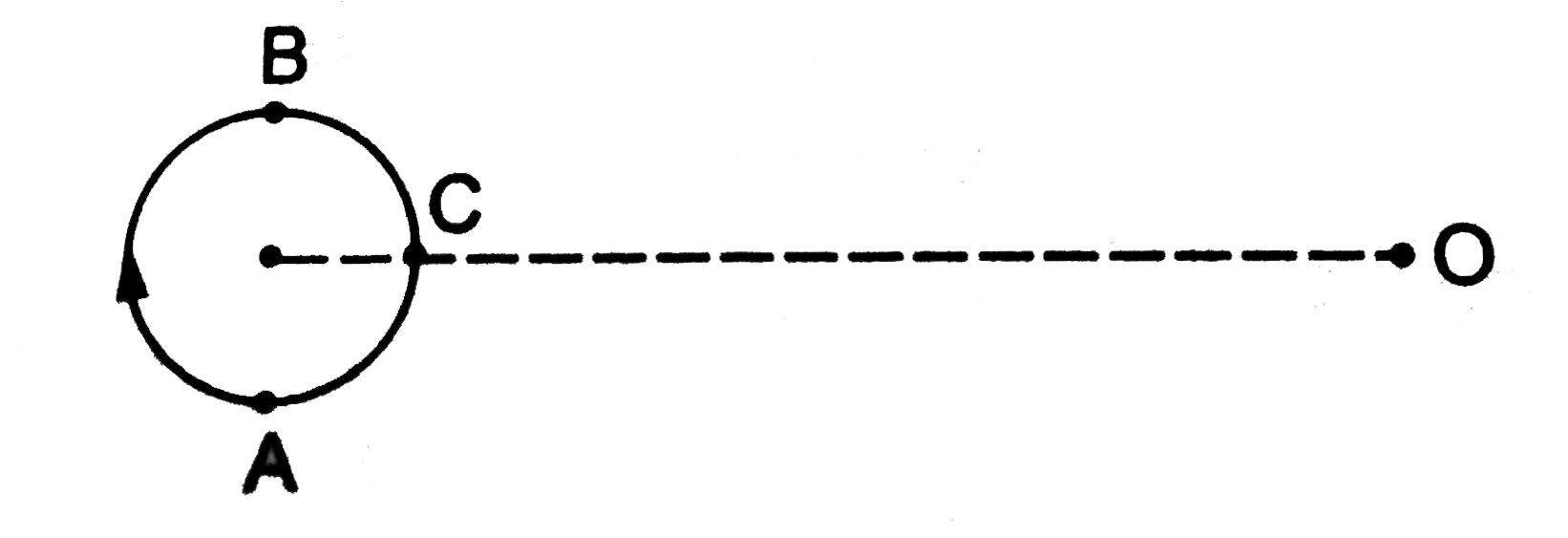# A small source of sound moves on a circle as shown in figure and an observer is sitting at O. Let v_1,v_2,v_3 be the frequencies heard when the sour

22 views
in Physics
A small source of sound moves on a circle as shown in figure and an observer is sitting at O. Let v_1,v_2,v_3 be the frequencies heard when the source is at A,B and C respectively..
A. v_1gtv_2ltv_3
B. v_1=v_2gtv_3
C. v_2gtv_3gtv_1
D. v_1gtv_3gtv_2

by (25.0k points)